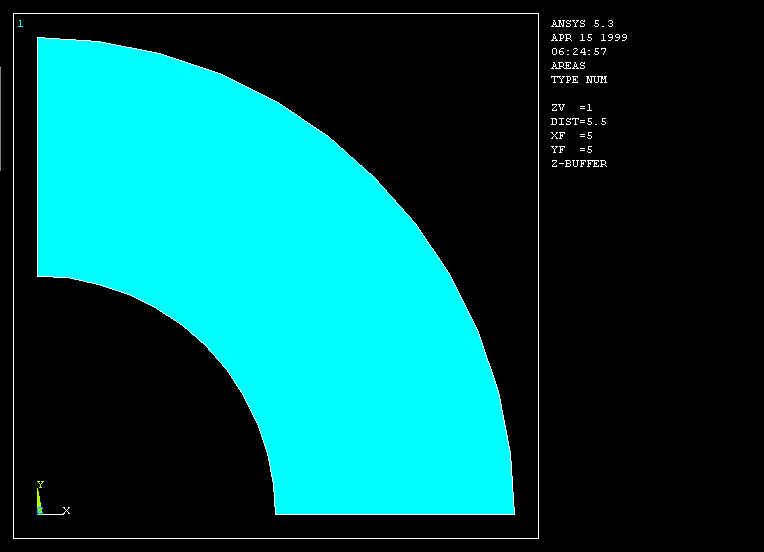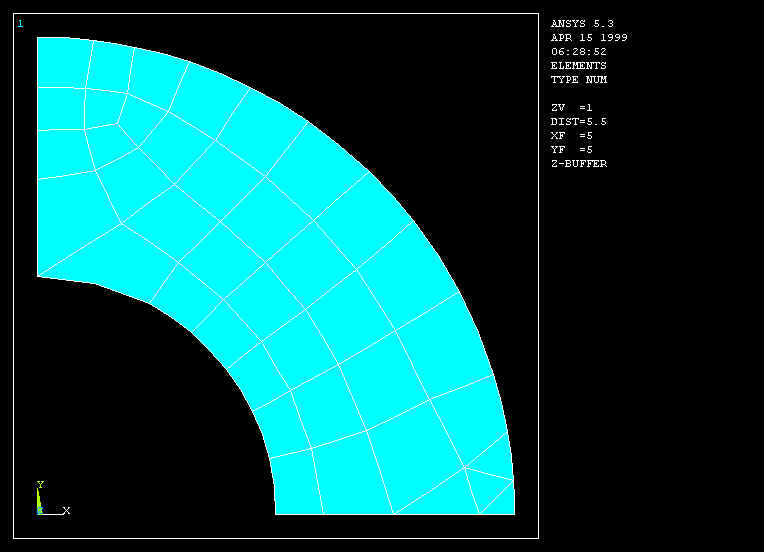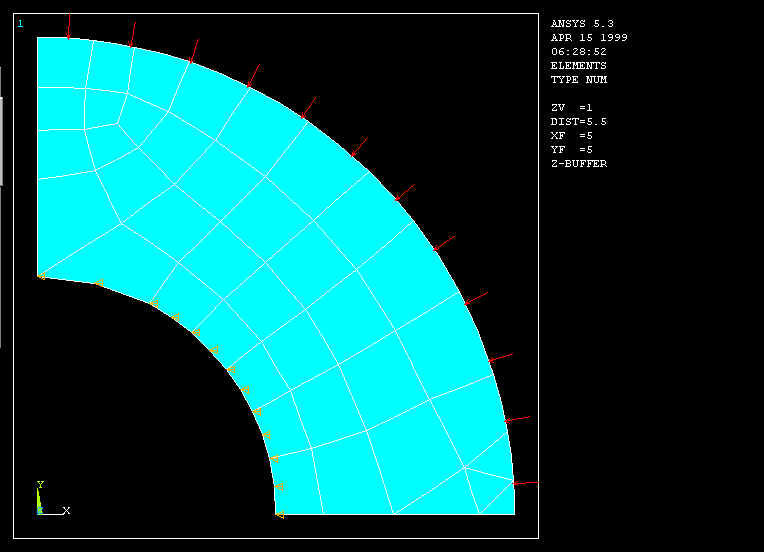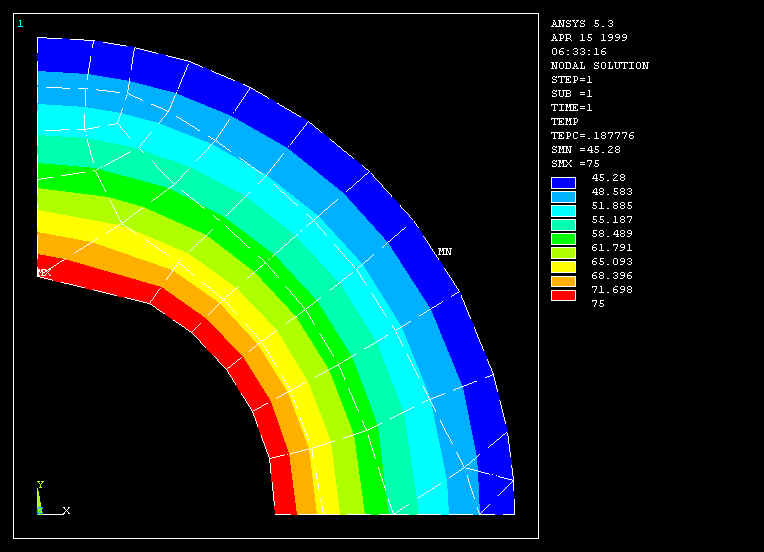Temperature distribution in a Cylinder

We wish to compute the temperature distribution in a long steel cylinder with inner radius 5 inches and outer radius 10 inches.  The interior of the cylinder is kept at 75 deg F, and heat is lost on the exterior by convection to a fluid whose temperature is 40 deg F.   The convection coefficient is 0.56 BTU/hr-sq.in-F and the thermal conductivity for steel is 0.69 BTU/hr-in-F.

1. Start ANSYS and assign a job name to the project.  Run Interactive -> set working directory and jobname.

2.  Preferences -> Thermal will show -> OK

3.  Recognize symmetry of the problem, and  a quadrant of a section through the cylinder is created using ANSYS area creation tools.  Preprocessor -> Modeling -> Create -> Areas -> Circle -> Partial annulusThe following geometry is created.4.  Preprocessor -> Element Type -> Add/Edit/Delete -> Add -> Thermal Solid -> Solid 8 node 77 -> OK -> Close

5.  Preprocessor -> Material Props -> Isotropic -> Material Number 1 -> OK

EX = 3.E7 (psi)

DENS = 7.36E-4 (lb sec^2/in^4)

ALPHAX = 6.5E-6

PRXY = 0.3

KXX = 0.69 (BTU/hr-in-F)

6. Mesh  the area and refine using methods discussed in previous examples.7.  Preprocessor -> Loads -> Apply -> Temperatures -> Nodes

Select the nodes on the interior and set the temperature to 75.

8.   Preprocessor -> Loads -> Apply -> Convection -> Lines

Select the lines defining the outer surface and set the convection coefficient to 0.56 and the fluid temp to 40.

9.  Preprocessor -> Loads -> Apply -> Heat Flux -> Lines

To account for symmetry, select the vertical and horizontal lines of symmetry and set the heat flux to zero.10.  Solution -> Solve current LS

11. General Postprocessor -> Plot Results -> Nodal Solution  -> TemperaturesThe temperature on the interior is 75 F and on the outside wall it is found to be 45.  These results can be checked using  results from heat transfer theory.

Back